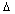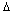Published 10.04.2003

PROSPECTS OF HYDROGEN ENERGETICS

Ph.M. Kanarev

E-mail: kanphil@mail.kuban.ru

Hydrogen is the only pollution free and inexhaustible energy carrier,  but the implementation of such attractive properties of hydrogen is restrained by large expenses of energy for its production from water. Modern level of knowledge allows us to implement this process and to reduce energy expenses for hydrogen production , , , .

There are the notions of  gram-atom and gram molecule in chemistry. Gram-atom is equal numerically  to atomic mass of the substance, gram molecule is equal numerically to molecular mass of the substance. For example, hydrogen gram molecule in water molecule  is equal to 2 grams, and gram-atom of oxygen atom is equal to 16 grams. Gram-molecule of water is equal to 18 grams. As the mass of hydrogen  in water molecule is 2×100/18=11.11% and the mass of oxygen atom  is 16×100/18=88.89%, the ratio between quantity of hydrogen and oxygen is preserved in one litre of water as well. It means that 1000 grams of one litre of water contain 111.1 grams of hydrogen molecules  and  888.9 grams of oxygen atoms.

One litre of molecular hydrogen  has mass of  0.09 grams, one litre of molecular oxygen  has mass of 1.47 grams. It means that from one litre of water it possible to produce 111.1/0.09=1234.44 litres  of  hydrogen   and 888.9/1.47=604.69 litres of oxygen.

Thus, one gram of water contains 1.23 litres of hydrogen. Existing energy consumption for production of 1 m3 of hydrogen from water is 4 kWh or  4Wh/liter of hydrogen. In order to produce 1.23 liters of  hydrogen,  it is necessary to spend 1.23x4=4.94 Wh or 4.94 Wh/gram of water.

Instruments and Equipment Used for the Experiment

The special experimental  low current electrolyser. The instruments used for input power measurement: an electric meter, voltmeter (accuracy class 0.2, GOST 8711-78), ammeter (accuracy class 0.2, GOST 871160). A balance with value of a division of  0.10 grams and 0.010 grams. A stopwatch with value of a division of 0.1 s.

Experimental Results

 Indices Average 1 - duration of the experiment with input energy in 6 series t, min 6x5=30.0 2 – readings of voltmeter V, volts 13.6 3 – ammeter readings I, amperes 0.02 4 – power P, watts hour (P=VxIxτ/60) 0.136 5 – continue of experiment without input energy in 6 series, min 6x55=330.0 6 - mass difference, grams 0.44 7 – mass of evaporated water, grams 0.02x6=0.12 8 – mass of water converted in hydrogenm, grams 0.320 9 – specific power P’=P/m, Watt/gram of water 0.425 10 – existing  specific power P’’, Watt/gram of water 4.94 11 – the reducing  power on the production of hydrogen, times K=P’’/P’ 11.62 12– quantity of released hydrogen, ΔМ =0.320x1.23x0.09=0.035, grams 0.035 13 – energy content of hydrogen being obtained      (Е=0.035х142/3,6) Wth 1.397 14- energy efficacy of low ampere process of water electrolysis (Eх100/P), % 1027

Note: Gas escape is clearly seen during many hours after the electrolyzer is disconnected from the supply line.

Conclusion

Low ampere water electrolysis opens a prospect for production of inexpensive hydrogen from water and a transit to hydrogen energetic.

References

1- Ph.M. Kanarev. The Foundation of Physchemistry of the Micro World. Krasnodar, 2002. 320 pages

3 – Ph. M. Kanarev. T. Mizuno. Cold Fusion by Plasma Electrolysis of Water. New Energy Technologies. 2003. Issue N 1 (10), pag. 5-10.

Webmaster J. Hartikka

http://Kanarev.hydrogen.innoplaza.net

<< Kanarev´s Page